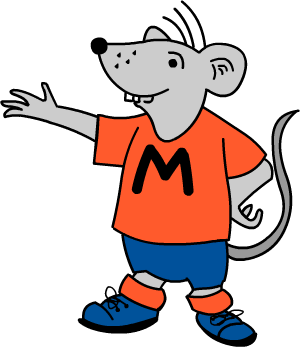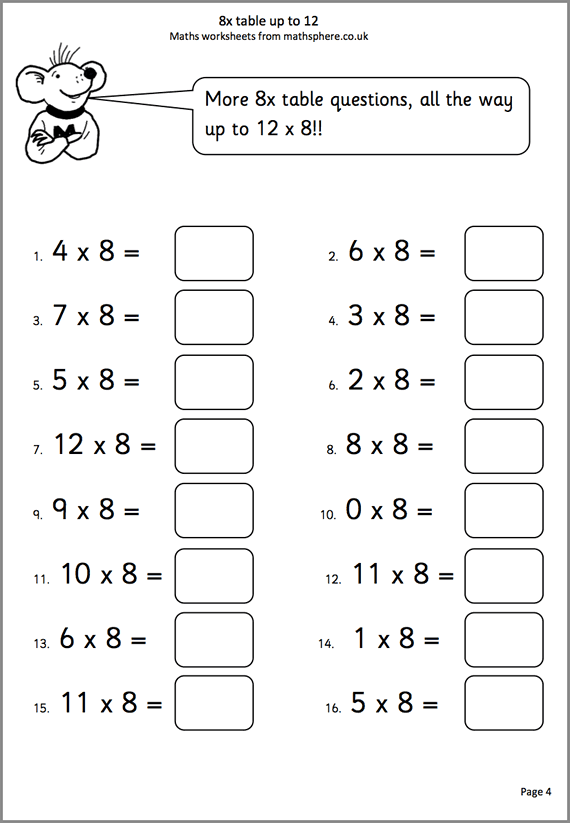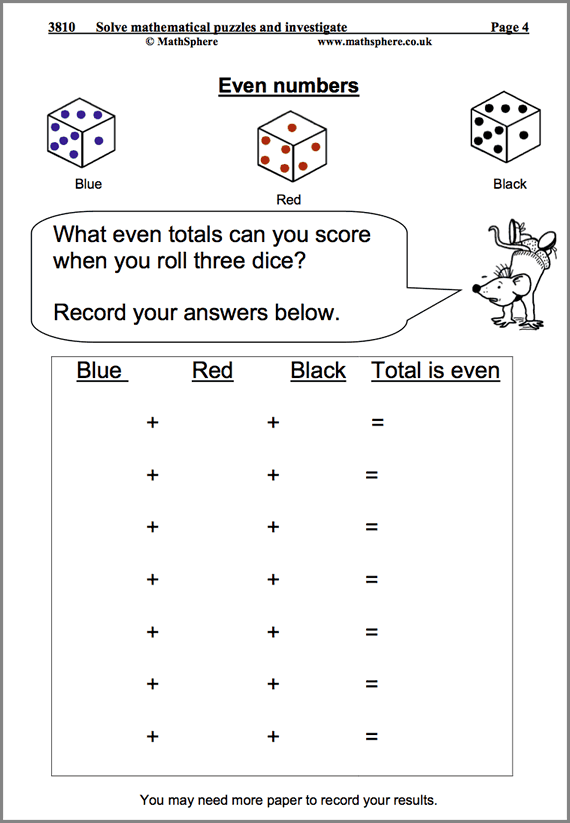# Year 3 Maths Worksheets

The Year 3 worksheets provide a really effective way to ensure children make good progress in Maths. This is an indispensable resource for any teacher following the new Programme of Study as well as providing superb reinforcement for maths at home.

## The Very Best Year 3 Maths Worksheets

Our Year 3 worksheets have been written to meet the high expectations for 7‐8 year olds, including new challenges such as mentally calculating with 3-digit numbers, learning the 8x table, fractions and using the 24-hour clock.

• Updated for the very latest statutory requirements with many new pages added
• Answers included to make marking simple
• Concept pages clearly explain the ideas being taught
• Over 1000 high quality pages of questions and answers
• Single user licence for teachers or parents (school site licence upgrade available)
• MathSphere has been providing software for schools for over 15 years## Try Some Free Sample Worksheets

To give you a better idea of the worksheets available we have provided a selection of free modules for Year 3.

Please download these samples, print them out and use at home, or photocopy and use in the classroom.6 Pages

#### 8 × Tables To 12

Plenty of help with learning the 8 times table up to 12 × 8.6 Pages

#### Short Division

Using the short division method, including remainders.23 Pages

#### Solve Maths Puzzles

Problems and puzzles to encourage a systematic approach to maths.

## List of modules

It is impossible to describe in a single page the massive amount of high quality maths contained in this one product.

To give you some idea, have a look at the list of all modules:

### Number and Place Value 156 pages

 Revise counting on and back. 18 Read and write 3-digit numbers. Partition into HTU. 15 Counting in tens and hundreds. Odd and even numbers. 19 Count in fours and eights. 7 Count in fifties. 6 Describe and extend number sequences. 14 Comparing and ordering numbers. 16 One, ten or one hundred more than or less than. 18 Order a set of familiar numbers. 9 Estimation and approximation. 11 Rounding a number to the nearest 10 and 100. 13 Further rounding. 10

### Multiplication and Division 173 pages

 Revise understanding of multiplication. 16 Know multiplication facts by heart. Relate to division. 8 3x and 4x tables up to 12. 13 8x table up to 12. 6 Tables: speed sheets. 21 Revise division. 15 Strategies for multiplication and division. 16 Multiply and divide by 10 and 100. Doubling and halving. 21 Understand remainders. Rounding after division. 15 Develop written methods for multiplication 6 Written methods for multiplication. 9 Written division using repeated subtraction. 6 Simple written division. 5 Formal method of short division. 6 Choose appropriate number operations. 10

### Measurement 133 pages

 Measure and compare. 17 Choose and use suitable units to estimate and measure. 7 Use measuring equipment . Interpret scales. 10 Solve problems involving money. 20 Measuring perimeter. 8 Solve problems involving measurement. 13 Understand and read the time. 16 Read the time from clocks, calendars and timetables. 26 Time: a.m. and p.m. and 24 hour clock. 9 Roman numerals and time. 7

### Statistics 33 pages

 Collect, sort and organise data. 10 Use bar charts, tally charts, bar graphs and pictograms. 15 Use Venn and Carroll diagrams. 8

### Mental arithmetic 75 pages

 Autumn term. 25 Spring term. 25 Summer term. 25

### Fractions 47 pages

 Recognise simple fractions and equivalence. 13 Recognise and find simple fractions. 12 Find unit fractions of numbers or quantities. 10 Adding fractions. 4 Subtracting fractions. 4 Ordering fractions. 4

### Geometry 67 pages

 Describe and classify 3D and 2D shapes. 12 Make shapes and describe their features. 12 Describe movement and understand angle as a measure of turn. 9 Describe position and direction. 9 Perpendicular and parallel lines. 7 Symmetry. 11 Pentominoes. 7

### Using and Applying Mathematics 104 pages

 Solve mathematical puzzles and investigate. 23 Investigate general statements. 10 Investigate addition. 11 Investigate subtraction. 10 Investigate multiplication. 4 Solve word problems. 16 Maths vocabulary word searches. 8 Investigate column addition. 4 Routes investigation. 5 Investigate coins. 4 More coins. 5 Add one to four. 4

### Quick Details

• Over 1000 worksheets